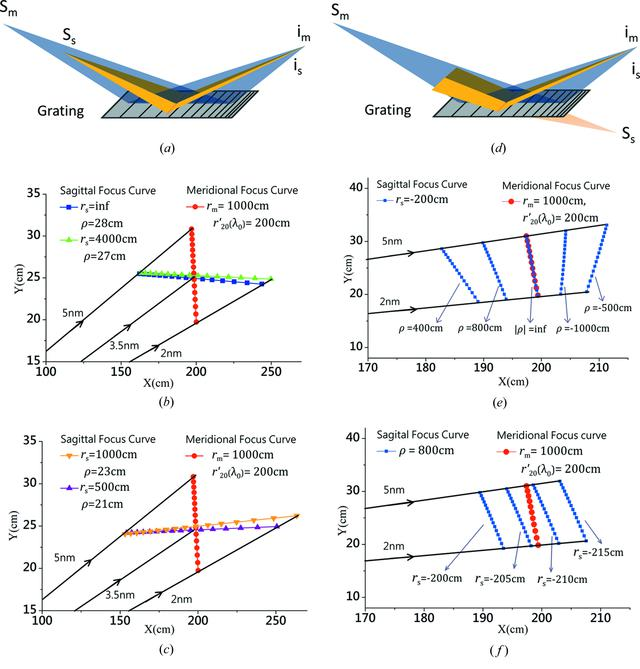disable zoom     view article Figure 3 Schematic diagrams to illustrate the light source with different object distances in its meridional (gray) or sagittal (yellow) coordinates, achieving the identical image focal length in the two directions. (a) Both the meridional and sagittal object distances are real (i.e.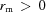and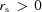). (d) The meridional object distance is real, while the sagittal object distance is virtual (i.e.and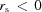). The symbols Sm or im represent the meridional object (or image) point, while Ss or is represent the sagittal object (or image) point. The optimal meridional focal curve (red spots) is presented in each diagram (b, c, e and f) as the control group, where rm = 1000 cm,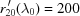cm (at the center wavelength), and the other parameters are the same as those for the optimal case in Fig. 1(R = 52729 cm). The sagittal focal curves for various cases are presented in specific plots for comparison. (b) The sagittal focal curves for rs = infinity,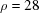cm and rs = 40 m,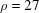cm. (c) The sagittal focal curve for rs = 10 m,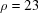cm and rs = 5 m,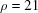cm. (e) The sagittal focus curves for fixing rs = -200 cm while changing. (f) The sagittal focus curves for fixing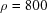cm while changing rs.JOURNAL OFSYNCHROTRONRADIATION
ISSN: 1600-5775
Volume 25| Part 3| May 2018| Pages 738-747